Exponents 5
Set 5 (10 Questions)
Determine the prime factors of all numbers through 50 and write the numbers as the product of their prime factors by using exponents to show multiples of a factor (e.g., 24 = 2 x 2 x 2 x 3 = two cubed times three)
From Mr. Anker Tests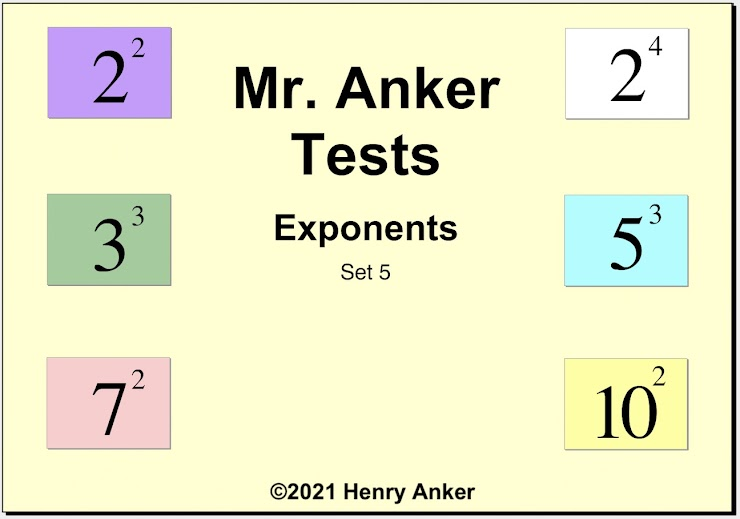Exponents Help Slide 1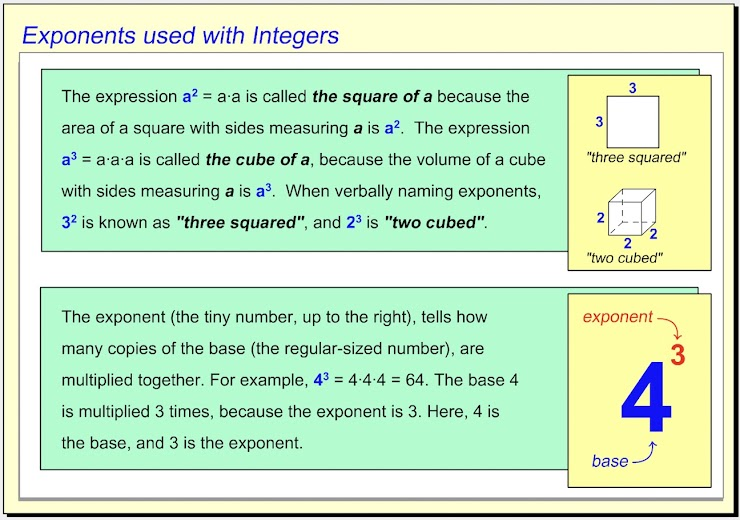Exponents Help Slide 2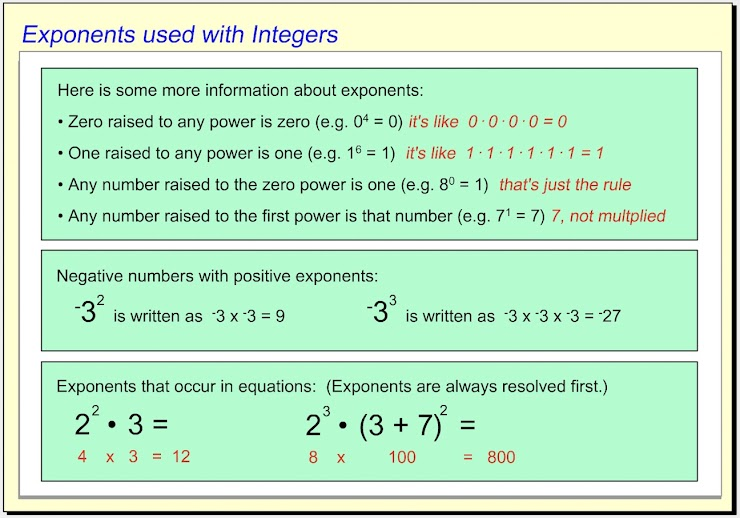Exponents Help Slide 3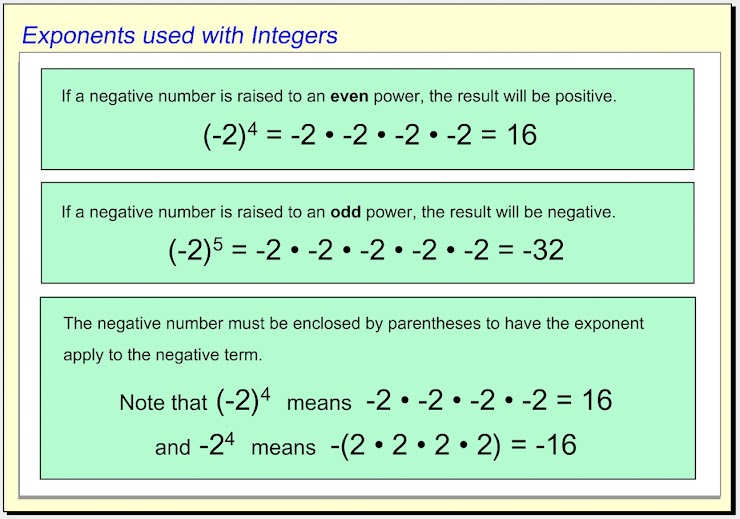1.  What is the value of a? *
1 point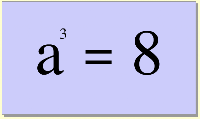2.  Use the dropdown menu to make this statement true:  "The quotient of eight divided by four, raised to the fifth power is __________ the quotient of twelve divided by two squared." *
1 point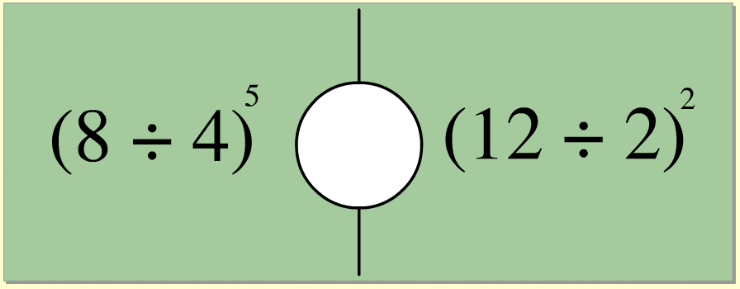3.  What is the value of a? *
1 point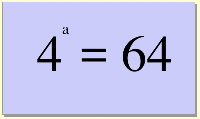4.  Use the dropdown menu to make this statement true:  "The difference of seven minus three squared is_________ the difference of five squared and ten." *
1 point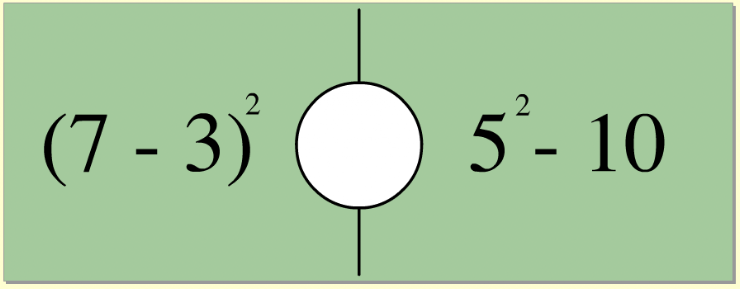5.  Which choice below is the correct way to name the integer with the exponent shown? *
1 point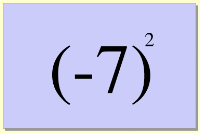6. Use the dropdown menu to make this statement true:  "Negative seven squared is ___________ seven squared." *
1 point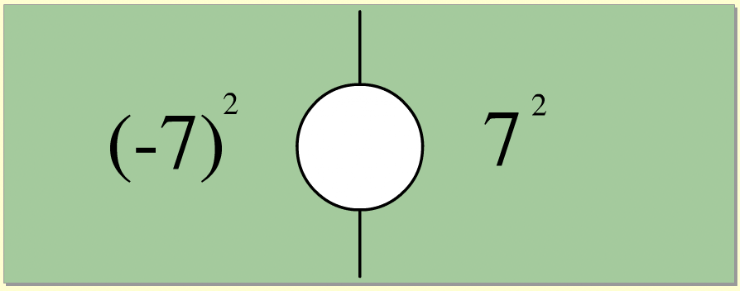7.  On the line below, enter the equal value as a whole number. *
1 point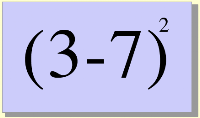8. Use the dropdown menu to make this statement true:  "Ten minus the difference of twelve minus four squared is ___________ the quotient of thirty-six divided by six squared." *
1 point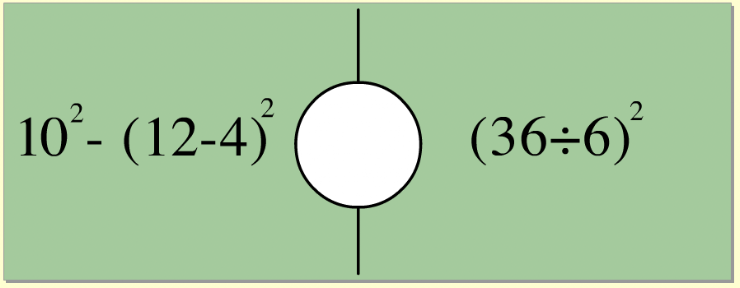9.  Which of the choices below is equal to the integer with the exponent shown? *
1 point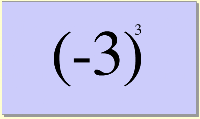10.   On the line below, enter the equal value as a whole number. *
1 point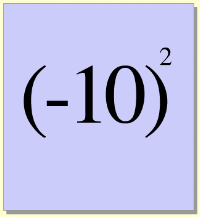Submit
Clear form